# Phase Portraits of Nonlinear Systems

Consider a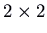, possibly nonlinear, autonomous system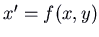,(autonomous means that the independent variable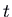, thought of as representing time, does not occur on the right sides of the equations). Just as we did for linear systems, we want to look at the trajectories of the system. Since in most cases it is impossible to solve these systems exactly, we will concentrate on the qualitative aspects, and in particular on how to sketch the phase portrait by hand.

Here are some of the principles of trajectory sketching:

• Trajectories follow the direction field. The velocity vector for a solution at a point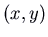in the plane is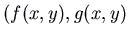. The direction of the trajectory is the direction of this vector.
• The curves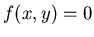and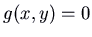are the isoclines on which the direction of a trajectory is vertical and horizontal respectively. These isoclines divide up the plane into regions. In each region, the signs of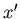and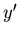don't change, so e.g. if both are positive the direction is always up and to the right. Typically as you cross an isocline, one component of the velocity changes sign. Thus by knowing the direction at one point you can determine the directions in all the regions.
• An isocline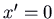(or part of it) that is a vertical line is a trajectory (or maybe several of them). Similarly, an isocline(or part of it) that is a horizontal line is a trajectory (or several).
• Trajectories don't meet or stop, except that in the limit as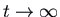or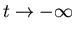they can approach an equilibrium point.
• The equilibrium points (also known as critical or stationary points) are the points where bothand. Thus they are at intersections of those isoclines.
• The behaviour near the equilibrium points is important. The trajectories near an equilibrium point are approximated very well by those of the linearization of the system at that point, and the critical point can be classified using that linearization (with two exceptions which we will see).
• A separatrix (plural separatrices) is a trajectory separating two regions in which the behaviour of solutions asoris different. The trajectories entering and leaving a saddle point are very often separatrices. These should be among the first trajectories you sketch.
• If the system has some symmetry, that may help. Examples of symmetry include interchanging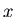and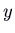, or changing the sign ofand/or.

The linear approximation to a function of two variables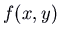near a point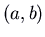is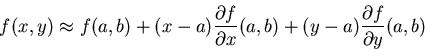We want to do this in particular at an equilibrium point, where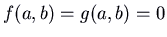. It is useful to make a change of variables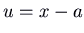,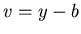, so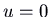,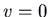corresponds to the equilibrium point. Then the linearization of our system at this equilibrium point is theconstant-coefficient linear homogeneous system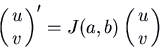where the matrix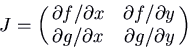is called the Jacobian of the vector field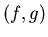.

Example: Consider the system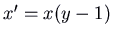,.

For an equilibrium point we need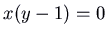(i.e.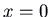or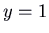) and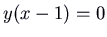(i.e.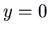or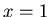). There are thus two equilibrium points: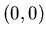and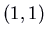.

Before classifying the equilibrium points, it's a good idea to draw the isoclines forand. I'll plot the first in blue and the second in green. I indicate with arrows the direction field in each region and on the isoclines. The intersections of the blue and green isoclines are the equilibrium points.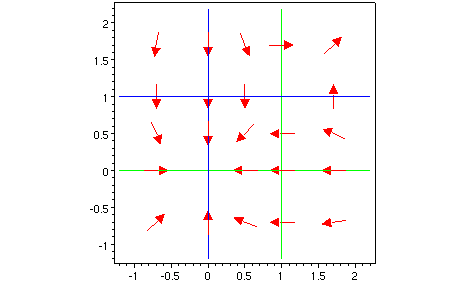The Jacobian matrix is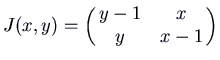. At the equilibrium pointthis is. That has a double eigenvalue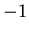, and two linearly independent eigenvectors. Therefore the equilibrium pointis a singular node, and is an attractor.

At the second equilibrium pointthe Jacobian matrix is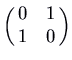. This has eigenvalues 1 and. Therefore the equilibrium pointis a saddle. The eigenvectors are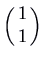for 1 and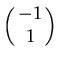for. The picture below shows the phase plane with some parts of trajectories near the two equilibrium points. Note that the directions of these trajectories agree with the direction field arrows from the previous picture.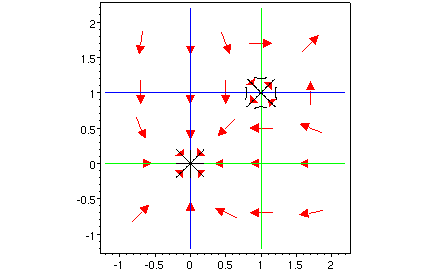Now we sketch some trajectories. There are trajectories on theandaxes (going inwards to the equilibrium point at the origin), becausewhenandwhen. Next it's a good idea to sketch the trajectories coming out of and going in to the saddle point. For example, one comes in to the saddle point from below and to the right. We go backwards in time, following the arrows backward. These arrows point up and left in the region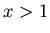,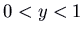. The trajectory must curve to avoid the trajectory on the positiveaxis. It is presumably asymptotic to that axis. The trajectory coming out of the saddle point down and to the left must continue down and to the left until it ends at the equilibrium point.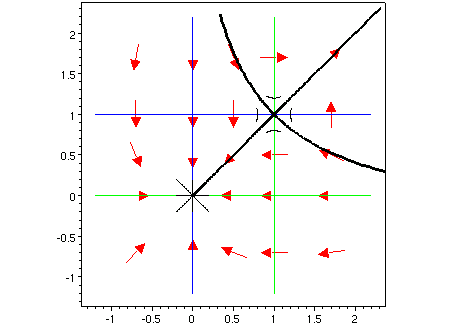Finally, we draw some more trajectories, including at least one in each region. Note that those trajectories that enter the equilibrium point atcan do so at any angle.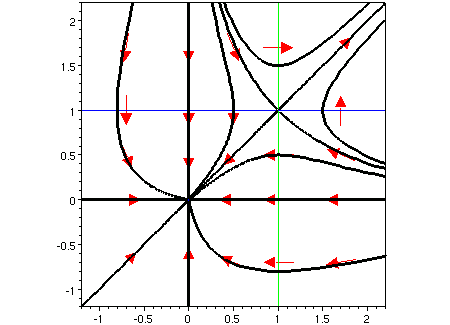Our picture is symmetric about the line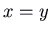, because of the fact that the system of equations remains the same if you interchangeand.

The trajectories entering and leaving the saddle point are separatrices. All trajectories below and to the left of the two trajectories entering the saddle go to the equilibrium pointas, while those above and to the right go off to infinity asymptotic to the line. Below and to the right of the trajectories leaving the saddle, everything comes in from infinity asymptotic to the positiveaxis (as), while above and to the left of these everything comes in from infinity asymptotic to the positiveaxis.

As I mentioned, there are two exceptions to the rule that the phase portrait near an equilibrium point can be classified by the linearization at that equilibrium point. The first is where 0 is an eigenvalue of the linearization (we didn't even look at the linear system in that case!). The second exception is where the linearization is a centre. The linear system has periodic solutions, corresponding to trajectories that are closed curves (ellipses). Imagine starting at some point and following the direction field. After going all the way around the equilibrium point, in the linear system you return exactly to the point you started at. This is a very delicate matter, and any nonlinear effect, even if very small, could spoil it. If in the nonlinear system you come back slightly farther away from the equilibrium point than where you started, then your trajectory can not be a closed curve. The next time around, you will be still farther away. The trajectory will spiral away from the equilibrium point. If all trajectories near the equilibrium point are like this, the equilibrium point is an unstable spiral. On the other hand, if after one turn around the equilibrium point you are slightly closer than where you started, your trajectory spirals inwards. If all trajectories near the equilibrium point are like this, the equilibrium point is a stable spiral (and an attractor). Here are pictures of those two possibilities. The third possibility, of course, is that you do come back exactly to the point where you started, and it really is a centre.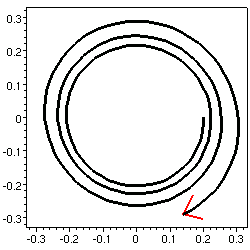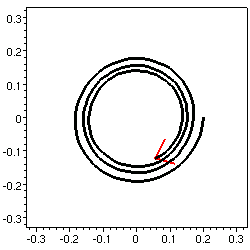One way to show that a centre of the linearized system is still a centre in the nonlinear system is to find an equation for the trajectories. If there is such an (implicit) equation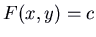where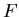is a smooth function, not constant in any region, and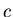an arbitrary constant, then the trajectories, which are level curves of this function, can not be spirals but can be closed curves. This occurs both in the predator-prey and pendulum systems.

Robert Israel
2002-04-01